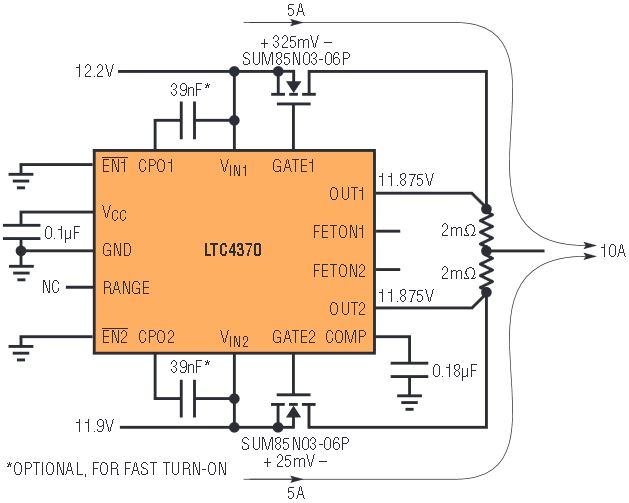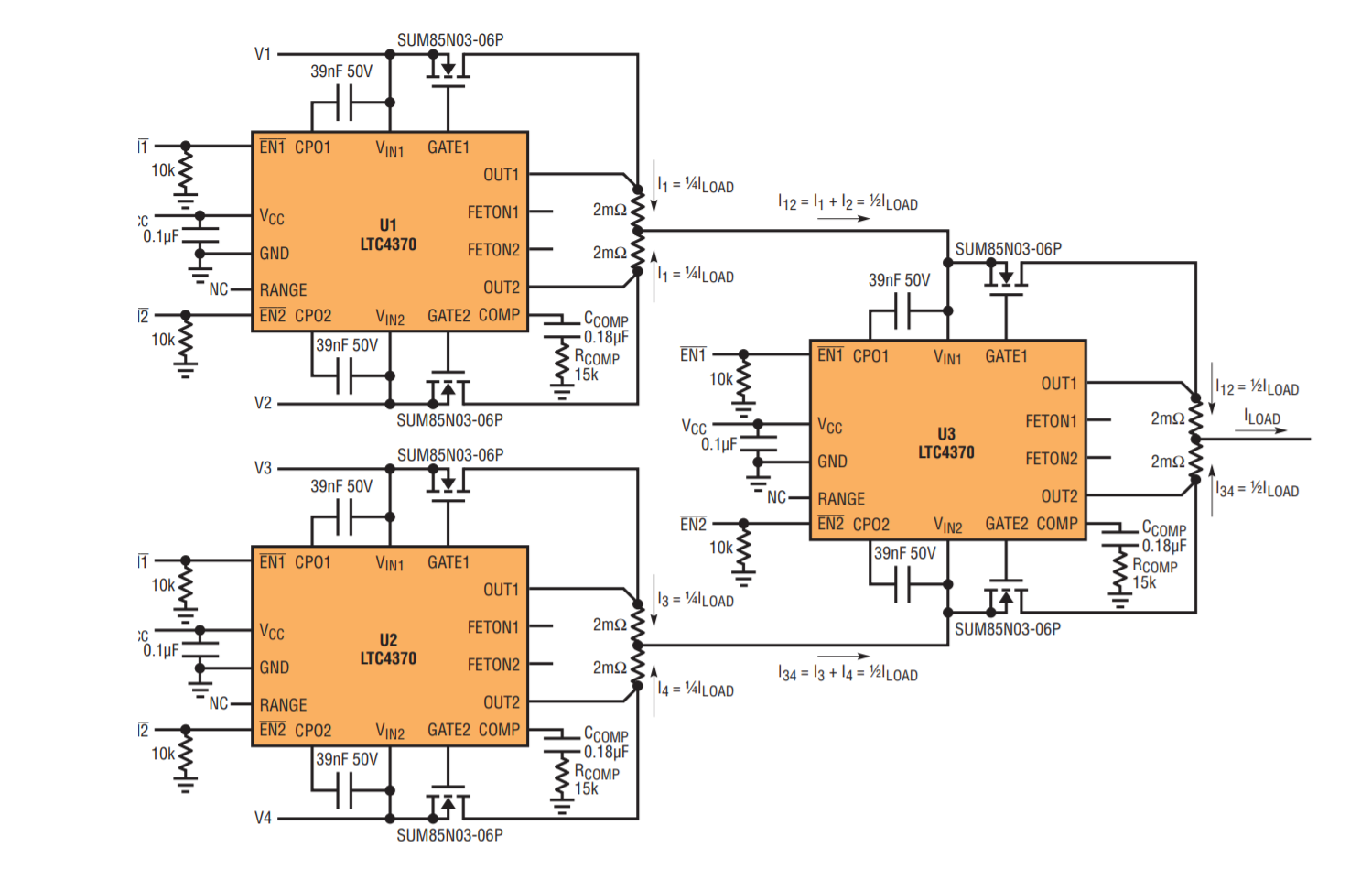# Easy Balanced Load Sharing for Three or Four Supplies, Even with Unequal Supply Voltages

Using multiple small power supplies is often more economical and more reliable than using a single large power supply. For instance, separate batteries can be used for higher reliability. In a multi-supply system, it is important that the load is equally shared; otherwise, one supply may attempt to carry the entire load. This article shows how to easily load balance three or four supplies by cascading LTC4370 circuits.

The LTC4370 controller enables current sharing between two supplies with a modest difference between the output voltages, as shown in Figure 1. To perfectly balance the current in both sides, the controller regulates the gate-source voltage of an N-channel MOSFET in whichever side has the higher voltage. This creates a voltage drop across the MOSFET’s RDS(ON) plus the current sense resistor.Figure 1. The LTC4370 current-balancing controller enables balanced load sharing between two supplies, even when their voltage outputs are different.

The LTC4370 can compensate for a voltage difference between two rails of up to 0.5V. If the voltage difference of the two supplies is somewhat less than 0.5V, the LTC4370 can regulate its output to match the lower value rail, set by adding an appropriate resistor on the RANGE pin.

Balancing the Load Between Three Supplies with Two Cascaded LTC4370s

Figure 2 shows a 3-input, 12V system delivering 10A. Notice that one LTC4370 (U1) performs equal current sharing between supplies V1 and V2, while the second LTC4370 (U2) implements a 2:1 relation between the output current of U1 and the current of a third supply, V3. Thus, each supply contributes one third of the total load current equally. The output voltage at the load is less than the minimum of the supply voltages V1, V2 and V3. Because there are two stages of cascading, it is possible to have as much as 1V difference between V3 and V1 or V2, if the difference between V1 and V2 is already at the 0.5V limit.Figure 2. Two LTC4370s can be cascaded to enable current sharing of three supplies.

Balancing the Load Between Four Supplies

Cascading three LTC4370 controllers (Figure 2) allows four supplies to share the load. In the first stage, U1 and U2 force equal sharing between a pair of supplies, where the output current of U1 is I12 = I1 + I2, and the output current of U2 is I34 = I3 + I4. A third LTC4370, the second stage, keeps I12 = I34. Thus, each supply contributes one fourth of the total load current. The two stages, as above, allow the possibility of as much as 1V difference between the four supply voltages.Figure 3. Four supplies can each support an equal share of a load by using three LTC4370s in a 2-stage cascade.

Limitations

The main error sources that affect perfect current sharing are:

• LTC4370 error amplifier input offset, ±2mV (maximum)
• Sense resistor tolerance, worst-case for 1% resistors is 2% overall.

Sharing error attributed to the error amplifier input offset decreases with increasing sense voltage, but power dissipation increases. For the simple LTC4370 circuit with two supplies, this error is expressed as an imbalance in the supplies’ sharing of current:

Using the worst-case errors, above, the error is:

For the circuit of Figure 2, where ideal load sharing means the load is distributed into ⅓ILOAD and ⅔ILOAD, it is easier to estimate the worst-case imbalance via an expression of the maximum and minimum current of each supply:

Conclusion

By cascading the shared output of one LTC4370 with another LTC4370, three or more supplies can be efficiently controlled to provide equal current to the load. With errors on the order of the sense resistor tolerance, the voltage drop is minimal.